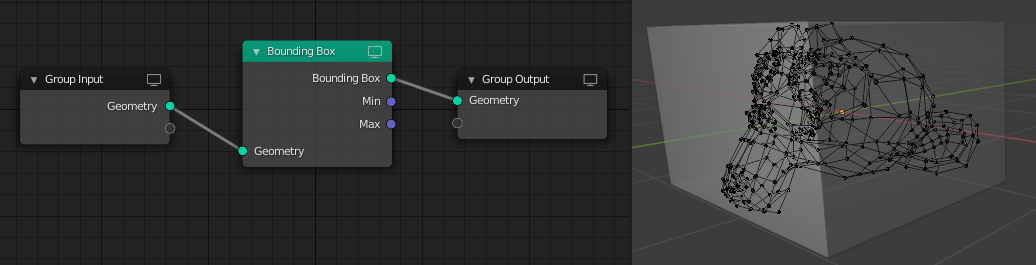# Bounding Box Node

The Bounding Box node creates a box mesh with the minimum volume that encapsulates the geometry of the input. The node also can output the vector positions of the bounding dimensions.

The mesh output and the Min and Max outputs do not take instances into account. Instead, for instanced geometry, a bounding box is computed for each instance rather than the whole geometry. To compute the bounding box including the instances, a Realize Instances Node can be used.

## Inputs

Geometry

Standard geometry input.

## Properties

This node has no properties.

## Outputs

Bounding Box

The resulting box that encapsulate the input geometry.

Min

The coordinates corresponding to the box’s -X, -Y, -Z position values, i.e. how far the box extends in each of the negative axes directions.

Max

The coordinates corresponding to the box’s +X, +Y, +Z position values, i.e. how far the box extends in each of the positive axes directions.

## ExampleBounding Box node used to create a box that encapsulates the geometry of the monkey mesh.